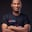Related Tags

javascript
communitycreator

# What are the every() and some() methods in JavaScript?Chibueze Sam-Obisike

In almost every JavaScript web application array, the methods filter(), map(), pop(), and push() are used. This article discusses two of these: the some() and every() methods.

These two methods are quite similar; however, they have a differentiating factor. After reading this shot, you should be able to understand and differentiate between some() and every().

### Array method some()

As the name implies, some() is a higher order test function that checks if the call back function passed into the some() argument is satisfied at least once. It returns a Boolean, true if the test is passed and false if it is not. However, it does not modify the value of the array.

#### Code

//Let us declare our Array

const arr=[1,2,3,4,5,6];

//Let us check if some elements in the array contains 2

let boolVal=arr.some(elemsInArr=>elemsInArr===2)
console.log(boolVal) //This returns a true


#### Explanation

In the code above:

1. We declared our array and called it arr.
2. We called the array method arr.some().
3. Then, we parsed in a callback function that picks every element in the arr and checks if any of the element equals 2.
4. We stored the value the arr.some() returns in a variable, called boolVal, and then console.log(boolVal), which returned true.

### Array method every()

Unlike the some() method, every() checks that every element in the given array pass the test within the callback function.

#### Code

Let’s check if every surveyor answered a question during their test, using the sample code below.

const surveyors = [
];

console.log(boolVal)

#### Explanation

Let’s analyze our code. We have an array of surveyors with an Object that has the name key and answer key.

We want to check if all of the surveyors answered a question.

We create a call back that loops through all the elements in the surveyors object and check if each surveyor answer’s surveyor.answer value pair equals "Yes".

If all the objects in the array satisfy the criteria, then surveyor.every() will return true. Otherwise, it will return false.

### Summary

The every() array method in JavaScript is used to check if all the elements of the array satisfy the callback function condition or not.

The some() array method in JavaScript is used to check if at least one of the elements passes the callback test or not.

RELATED TAGS

javascript
communitycreator

CONTRIBUTORChibueze Sam-Obisike
RELATED COURSES

View all Courses

Keep Exploring

Learn in-demand tech skills in half the time